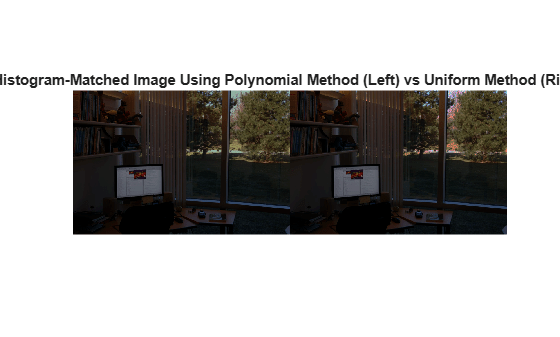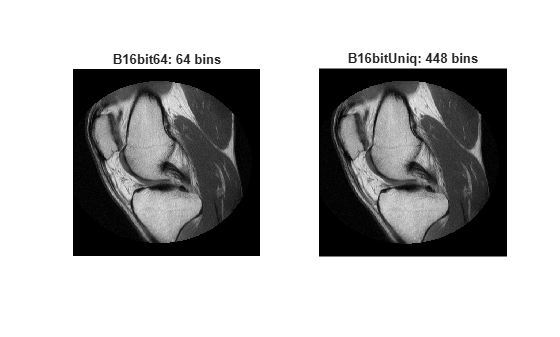# imhistmatch

Adjust histogram of 2-D image to match histogram of reference image

## Syntax

``J = imhistmatch(I,ref)``
``J = imhistmatch(I,ref,nbins)``
``J = imhistmatch(___,Name,Value)``
``````[J,hgram] = imhistmatch(___)``````

## Description

example

````J = imhistmatch(I,ref)` transforms the 2-D grayscale or truecolor image `I` returning output image `J` whose histogram approximately matches the histogram of the reference image `ref`.If both `I` and `ref` are truecolor images, then `imhistmatch` matches each color channel of `I` independently to the corresponding color channel of `ref`.If `I` is a truecolor RGB image and `ref` is a grayscale image, then `imhistmatch` matches each channel of `I` against the single histogram derived from `ref`.If `I` is a grayscale image, then `ref` must also be a grayscale image.Images `I` and `ref` can be any of the permissible data types and need not be equal in size.```

example

````J = imhistmatch(I,ref,nbins)` uses `nbins` equally spaced bins within the appropriate range for the given image data type. The returned image `J` has no more than `nbins` discrete levels. If the data type of the image is either `single` or `double`, then the histogram range is [0, 1].If the data type of the image is `uint8`, then the histogram range is [0, 255].If the data type of the image is `uint16`, then the histogram range is [0, 65535].If the data type of the image is `int16`, then the histogram range is [-32768, 32767]. ```

example

````J = imhistmatch(___,Name,Value)` uses name-value pairs to change the behavior of the histogram matching algorithm.```

example

``````[J,hgram] = imhistmatch(___)``` returns the histogram of the reference image `ref` used for matching in `hgram`. `hgram` is a 1-by-`nbins` (when `ref` is grayscale) or a 3-by-`nbins` (when `ref` is truecolor) matrix, where `nbins` is the number of histogram bins. Each row in `hgram` stores the histogram of a single color channel of `ref`.```

## Examples

collapse all

These aerial images, taken at different times, represent overlapping views of the same terrain in Concord, Massachusetts. This example demonstrates that input images `A` and `Ref` can be of different sizes and image types.

Load an RGB image and a reference grayscale image.

```A = imread('concordaerial.png'); Ref = imread('concordorthophoto.png');```

Get the size of `A`.

`size(A)`
```ans = 1×3 2036 3060 3 ```

Get the size of `Ref`.

`size(Ref)`
```ans = 1×2 2215 2956 ```

Note that image `A` and `Ref` are different in size and type. Image `A` is a truecolor RGB image, while image `Ref` is a grayscale image. Both images are of data type `uint8`.

Generate the histogram matched output image. The example matches each channel of `A` against the single histogram of `Ref`. Output image `B` takes on the characteristics of image `A` - it is an RGB image whose size and data type is the same as image `A`. The number of distinct levels present in each RGB channel of image `B` is the same as the number of bins in the histogram built from grayscale image `Ref`. In this example, the histogram of `Ref` and `B` have the default number of bins, 64.

`B = imhistmatch(A,Ref);`

Display the RGB image `A`, the reference image `Ref`, and the histogram matched RGB image `B`. The images are resized before display.

```imshow(A) title('RGB Image with Color Cast')``````imshow(Ref) title('Reference Grayscale Image')``````imshow(B) title('Histogram Matched RGB Image')```Read a color image and a reference image. To demonstrate the polynomial method, assign the reference image to be the darker of the two images.

```I = imread('office_4.jpg'); ref = imread('office_2.jpg'); montage({I,ref}) title('Input Image (Left) vs Reference Image (Right)');```Use the polynomial method to adjust the intensity of image `I` so that it matches the histogram of reference image `ref`. For comparison, also adjust the intensity of image `I` using the uniform method.

```J = imhistmatch(I,ref,'method','polynomial'); K = imhistmatch(I,ref,'method','uniform'); montage({J,K}) title('Histogram-Matched Image Using Polynomial Method (Left) vs Uniform Method (Right)');```The histogram-matched image using the uniform method introduces false colors in the sky and road. The histogram-matched image using the polynomial method does not exhibit this artifact.

This example shows how you can vary the number of bins in the target histogram to improve histogram equalization.

Load two images of data type `uint8` into the workspace. The images were taken with a digital camera and represent two different exposures of the same scene. `A` is an underexposed image and appears dark. `ref` is a reference image with good exposure and brightness.

```A = imread('office_2.jpg'); ref = imread('office_4.jpg');```

Display the images in a montage.

```montage({A,ref}) title('Dark Image (Left) and Reference Image (Right)')```Display the histogram of each color channel using 256 bins. You can use the helper function, `displayHistogramChannels`, that is included with the example.

`displayHistogramChannels(A,ref)`Image `A`, being the darker image, has most of its pixels in the lower bins. The reference image, r`ef`, fully populates all 256 bins values in all three RGB channels.

Count the number of unique 8-bit level values for each color channel of the dark and reference image. You can use the helper function, `countUniqueValues`, that is included with the example.

```numVals = countUniqueValues(A,ref); table(numVals(:,1),numVals(:,2),numVals(:,3), ... 'VariableNames',["Red" "Green" "Blue"], ... 'RowNames',["A" "ref"])```
```ans=2×3 table Red Green Blue ___ _____ ____ A 205 193 224 ref 256 256 256 ```

Equalize the histogram of the dark image using three different values of `nbins`: 64, 128 and 256. 64 is the default number of bins and 256 is the maximum number of bins for `uint8` pixel data.

```[B64,hgram64] = imhistmatch(A,ref,64); [B128,hgram128] = imhistmatch(A,ref,128); [B256,hgram256] = imhistmatch(A,ref,256); figure montage({B64,B128,B256},'Size',[1 3]) title('Output Image B64 | Output Image B128 | Output Image B256')```Display the histogram of each color channel using 256 bins. You can use the helper function, `displayThreeHistogramChannels`, that is included with the example.

`displayThreeHistogramChannels(B64,B128,B256)`Count the number of unique 8-bit level values for each color channel of the three histogram equalized images. As `nbins` increases, the number of levels in each RGB channel of output image `B` also increases.

```numVals = countUniqueValues(B64,B128,B256); table(numVals(:,1),numVals(:,2),numVals(:,3), ... 'VariableNames',["Red" "Green" "Blue"], ... 'RowNames',["B64" "B128" "B256"])```
```ans=3×3 table Red Green Blue ___ _____ ____ B64 57 60 58 B128 101 104 104 B256 134 135 136 ```

This example shows how to perform histogram matching with different numbers of bins.

Load a 16-bit DICOM image of a knee imaged via MRI.

```K = dicomread('knee1.dcm'); % read in original 16-bit image LevelsK = unique(K(:)); % determine number of unique code values disp(['image K: ',num2str(length(LevelsK)),' distinct levels']);```
```image K: 448 distinct levels ```
`disp(['max level = ' num2str( max(LevelsK) )]);`
```max level = 473 ```
`disp(['min level = ' num2str( min(LevelsK) )]);`
```min level = 0 ```

All 448 discrete values are at low code values, which causes the image to look dark. To rectify this, scale the image data to span the entire 16-bit range of [0, 65535].

```Kdouble = double(K); % cast uint16 to double kmult = 65535/(max(max(Kdouble(:)))); % full range multiplier Ref = uint16(kmult*Kdouble); % full range 16-bit reference image```

Darken the reference image `Ref` to create an image `A` that can be used in the histogram matching operation.

```%Build concave bow-shaped curve for darkening |Ref|. ramp = [0:65535]/65535; ppconcave = spline([0 .1 .50 .72 .87 1],[0 .025 .25 .5 .75 1]); Ybuf = ppval( ppconcave, ramp); Lut16bit = uint16( round( 65535*Ybuf ) ); % Pass image |Ref| through a lookup table (LUT) to darken the image. A = intlut(Ref,Lut16bit);```

View the reference image `Ref` and the darkened image `A`. Note that they have the same number of discrete code values, but differ in overall brightness.

```subplot(1,2,1) imshow(Ref) title('Ref: Reference Image') subplot(1,2,2) imshow(A) title('A: Darkened Image');```Generate histogram-matched output images using histograms with different number of bins. First use the default number of bins, 64. Then use the number of values present in image `A`, 448 bins.

```B16bit64 = imhistmatch(A(:,:,1),Ref(:,:,1)); % default: 64 bins N = length(LevelsK); % number of unique 16-bit code values in image A. B16bitUniq = imhistmatch(A(:,:,1),Ref(:,:,1),N);```

View the results of the two histogram matching operations.

```figure subplot(1,2,1) imshow(B16bit64) title('B16bit64: 64 bins') subplot(1,2,2) imshow(Ref) title(['B16bitUniq: ',num2str(N),' bins'])```## Input Arguments

collapse all

Input image to be transformed, specified as a 2-D truecolor or grayscale image. The returned image will take the data type class of the input image.

Data Types: `single` | `double` | `int16` | `uint8` | `uint16`

Reference image whose histogram is the reference histogram, specified as a 2-D truecolor or grayscale image. The reference image provides the equally spaced `nbins` bin reference histogram which output image `J` is trying to match.

Data Types: `single` | `double` | `int16` | `uint8` | `uint16`

Number of equally spaced bins in reference histogram, specified as a positive integer. In addition to specifying the number of equally spaced bins in the histogram for image `ref`, `nbins` also represents the upper limit of the number of discrete data levels present in output image `J`.

Data Types: `double`

### Name-Value Arguments

Specify optional comma-separated pairs of `Name,Value` arguments. `Name` is the argument name and `Value` is the corresponding value. `Name` must appear inside quotes. You can specify several name and value pair arguments in any order as `Name1,Value1,...,NameN,ValueN`.

Example: `imhistmatch(I,ref,'Method','polynomial')` matches the histogram of image `I` to that of reference image `ref` using the polynomial mapping technique.

Mapping technique used to map the histogram of `ref` to image `I`, specified as the comma-separated pair consisting of `'Method'` and one of these values:

• `'uniform'` — Use a histogram-based intensity function and histogram equalization.

• `'polynomial'` — Calculate a cubic Hermite polynomial mapping function from the cumulative histograms of the source and reference images. The polynomial method is useful when the reference image is darker than the input image. In this situation, the polynomial method gives a smoother color transition than the uniform method.

## Output Arguments

collapse all

Output image, returned as a 2-D truecolor or grayscale image. The output image is derived from image `I` whose histogram is an approximate match to the histogram of input image `ref` built with `nbins` equally-spaced bins. Image `J` is of the same size and data type as input image `I`. Input argument `nbins` represents the upper limit of the number of discrete levels contained in image `J`.

Data Types: `single` | `double` | `int16` | `uint8` | `uint16`

Histogram counts derived from reference image `ref`, specified as a vector or matrix. When `ref` is a truecolor image, `hgram` is a 3-by-`nbins` matrix. When `ref` is a grayscale image, `hgram` is a 1-by-`nbins` vector.

Data Types: `double`

## Algorithms

The objective of `imhistmatch` is to transform image `I` such that the histogram of image `J` matches the histogram derived from image `ref`. It consists of `nbins` equally spaced bins which span the full range of the image data type. A consequence of matching histograms in this way is that `nbins` also represents the upper limit of the number of discrete data levels present in image `J`.

An important behavioral aspect of this algorithm to note is that as `nbins` increases in value, the degree of rapid fluctuations between adjacent populated peaks in the histogram of image `J` tends to increase. This can be seen in the following histogram plots taken from the 16–bit grayscale MRI example.An optimal value for `nbins` represents a trade-off between more output levels (larger values of `nbins`) while minimizing peak fluctuations in the histogram (smaller values of `nbins`).